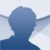# Cymath Android

2.44

## Have you ever been stuck on a math problem? The Cymath application offers the solutions to mathematical problems and the steps to obtain the answer

Can you imagine if there was a program that could solve all kinds of complex mathematical operations instantly? Well, not only does it exist, but it is completely free. But how does it work?

### The solution to all (mathematical) problems

Cymath is an educational tool that lets us solve mathematical problems and operations in seconds. And also, it shows us the step-by-step solution so that we can learn how to solve them ourselves.

The application to solve mathematical problems.

To use this app, we have two options: enter the operation with the calculator or take a picture of the math problem. The app will recognize the letters and numbers (even if they are handwritten) and will offer the answer and the steps to get there instantly.

With this app, we can differentiate, expand, factor, integrate, simplify, solve equations, and even create graphs. It also includes algebra and calculus problems to practice, as well as a blog with weekly problems that invite us to refresh our mathematical knowledge.

Equation solving, factoring, logarithms, exponents, complex numbers, quadratic equations, trigonometry, partial fraction, polynomial division, and more.

Whether we need help with our math homework or just want to solve a tricky operation, this practical and convenient app offers an excellent service through an elegant interface. All we have to do is download the free APK file.